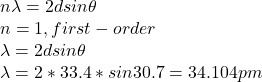Question

An x-ray beam of a certain wavelength is incident on a crystal, at 30.7° to a certain family of reflecting planes of spacing 33.4 pm. If the reflection from those planes is of the first order, what is the wavelength of the x rays?

1.Thunguyet

The wavelength of the x rays is 34.104 pm

Explanation:

Given data:

θ = incidence angle = 30.7°

d = space between planes = 33.4 pm = 33.4 x10⁻¹²m

According Bragg’s expression:2.niczorrrr

λ=34.068pm

Explanation:

Wavelength λ=2dsinθ

λ=2(33.4pm)sin30.7

λ=2(33.4pm) * 0.510

λ=66.8* sin30.7

λ=66.8*0.510

λ=34.068pm

X-rays are usually produced by charged particles that are accelerating or decelerating such as a beam of electrons striking a metal plate in an X-ray tube.